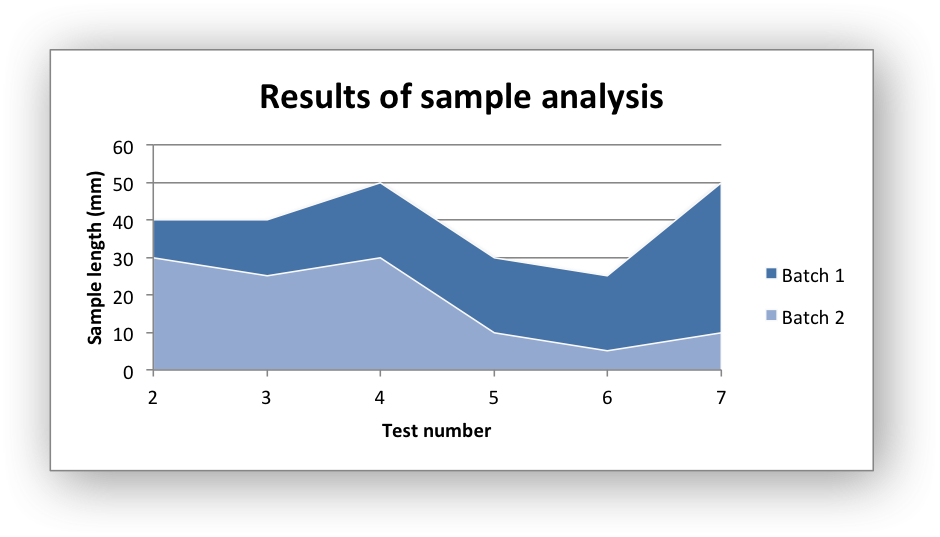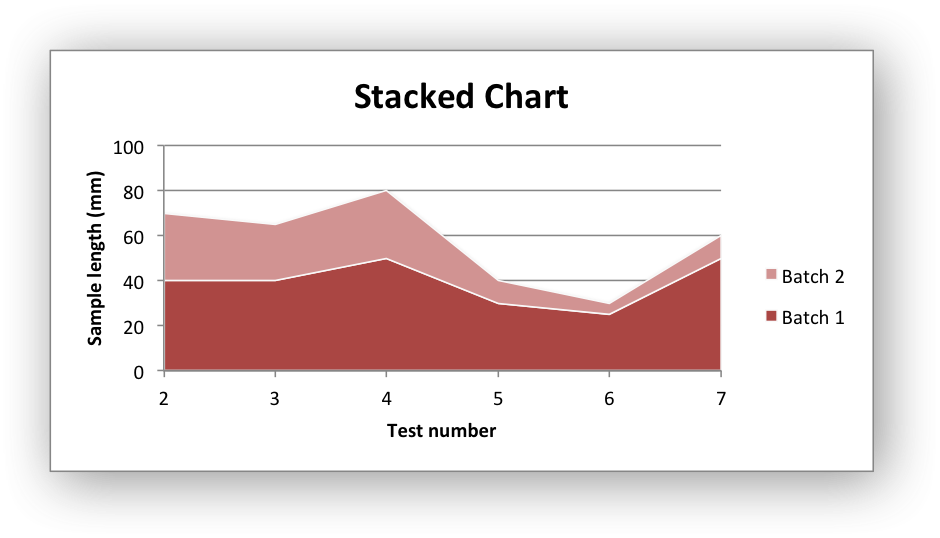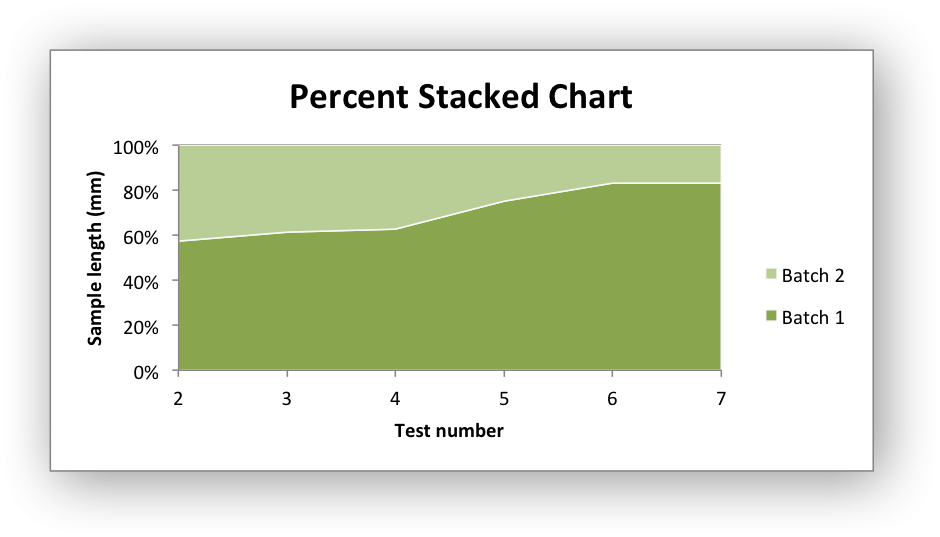# Example: Area Chart#

Example of creating Excel Area charts.

Chart 1 in the following example is a default area chart:Chart 2 is a stacked area chart:Chart 3 is a percentage stacked area chart:```#######################################################################
#
# An example of creating Excel Area charts with Python and XlsxWriter.
#
# Copyright 2013-2023, John McNamara, jmcnamara@cpan.org
#
import xlsxwriter

workbook = xlsxwriter.Workbook("chart_area.xlsx")

# Add the worksheet data that the charts will refer to.
headings = ["Number", "Batch 1", "Batch 2"]
data = [
[2, 3, 4, 5, 6, 7],
[40, 40, 50, 30, 25, 50],
[30, 25, 30, 10, 5, 10],
]

worksheet.write_column("A2", data)
worksheet.write_column("B2", data)
worksheet.write_column("C2", data)

#######################################################################
#
# Create an area chart.
#

# Configure the first series.
{
"name": "=Sheet1!\$B\$1",
"categories": "=Sheet1!\$A\$2:\$A\$7",
"values": "=Sheet1!\$B\$2:\$B\$7",
}
)

# Configure a second series. Note use of alternative syntax to define ranges.
{
"name": ["Sheet1", 0, 2],
"categories": ["Sheet1", 1, 0, 6, 0],
"values": ["Sheet1", 1, 2, 6, 2],
}
)

# Add a chart title and some axis labels.
chart1.set_title({"name": "Results of sample analysis"})
chart1.set_x_axis({"name": "Test number"})
chart1.set_y_axis({"name": "Sample length (mm)"})

# Set an Excel chart style.
chart1.set_style(11)

# Insert the chart into the worksheet (with an offset).
worksheet.insert_chart("D2", chart1, {"x_offset": 25, "y_offset": 10})

#######################################################################
#
# Create a stacked area chart sub-type.
#
chart2 = workbook.add_chart({"type": "area", "subtype": "stacked"})

# Configure the first series.
{
"name": "=Sheet1!\$B\$1",
"categories": "=Sheet1!\$A\$2:\$A\$7",
"values": "=Sheet1!\$B\$2:\$B\$7",
}
)

# Configure second series.
{
"name": "=Sheet1!\$C\$1",
"categories": "=Sheet1!\$A\$2:\$A\$7",
"values": "=Sheet1!\$C\$2:\$C\$7",
}
)

# Add a chart title and some axis labels.
chart2.set_title({"name": "Stacked Chart"})
chart2.set_x_axis({"name": "Test number"})
chart2.set_y_axis({"name": "Sample length (mm)"})

# Set an Excel chart style.
chart2.set_style(12)

# Insert the chart into the worksheet (with an offset).
worksheet.insert_chart("D18", chart2, {"x_offset": 25, "y_offset": 10})

#######################################################################
#
# Create a percent stacked area chart sub-type.
#
chart3 = workbook.add_chart({"type": "area", "subtype": "percent_stacked"})

# Configure the first series.
{
"name": "=Sheet1!\$B\$1",
"categories": "=Sheet1!\$A\$2:\$A\$7",
"values": "=Sheet1!\$B\$2:\$B\$7",
}
)

# Configure second series.
{
"name": "=Sheet1!\$C\$1",
"categories": "=Sheet1!\$A\$2:\$A\$7",
"values": "=Sheet1!\$C\$2:\$C\$7",
}
)

# Add a chart title and some axis labels.
chart3.set_title({"name": "Percent Stacked Chart"})
chart3.set_x_axis({"name": "Test number"})
chart3.set_y_axis({"name": "Sample length (mm)"})

# Set an Excel chart style.
chart3.set_style(13)

# Insert the chart into the worksheet (with an offset).
worksheet.insert_chart("D34", chart3, {"x_offset": 25, "y_offset": 10})

workbook.close()
```